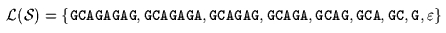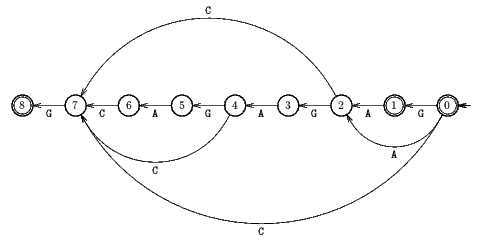Name
• String
• Substring
• Search
Edit

The Boyer-Moore type algorithms match some suffixes of the pattern but it is possible to match some prefixes of the pattern by scanning the character of the window from right to left and then improve the length of the shifts. This is made possible by the use of the smallest suffix automaton (also called DAWG for Directed Acyclic Word Graph) of the reverse pattern. The resulting algorithm is called the Reverse Factor algorithm.

The smallest suffix automaton of a word w is a Deterministic Finite Automaton S(w) =(Q,q0,T,E). The language accepted by S(w) is L(S(w))={u in* :  exists v in* such that w=vu}. The preprocessing phase of the Reverse Factor algorithm consists in computing the smallest suffix automaton for the reverse pattern xR. It is linear in time and space in the length of the pattern.

During the searching phase, the Reverse Factor algorithm parses the characters of the window from right to left with the automaton S(xR), starting with state q0. It goes until there is no more transition defined for the current character of the window from the current state of the automaton. At this moment it is easy to know what is the length of the longest prefix of the pattern which has been matched: it corresponds to the length of the path taken in S(xR) from the start state q0 to the last final state encountered. Knowing the length of this longest prefix, it is trivial to compute the right shift to perform.

The Reverse Factor algorithm has a quadratic worst case time complexity but it is optimal in average. It performs On.log(m) / m ) inspections of text characters on the average reaching the best bound shown by Yao in 1979.

Main features:

• uses the suffix automaton of xR;
• fast on practice for long pattens and small alphabets;
• preprocessing phase in O(m) time and space complexity;
• searching phase in O(mn) time complexity;
• optimal in the average.

Example:

Preprocessing phaseSuffix automaton used by Reverse Factor algorithm.

Searching phase

 G C A T C G C A G A G A G T A T A C A G T A C G * 8 7 2 G C A G A G A G

Shift by: 5 (8 - 3)

 G C A T C G C A G A G A G T A T A C A G T A C G * 8 7 6 5 4 3 2 1 - G C A G A G A G

Shift by: 7 (8 - 1)

 G C A T C G C A G A G A G T A T A C A G T A C G * 7 2 1 G C A G A G A G

Shift by: 7 (8 - 1)

The Reverse Factor algorithm performs 17 character comparisons on the example.

C